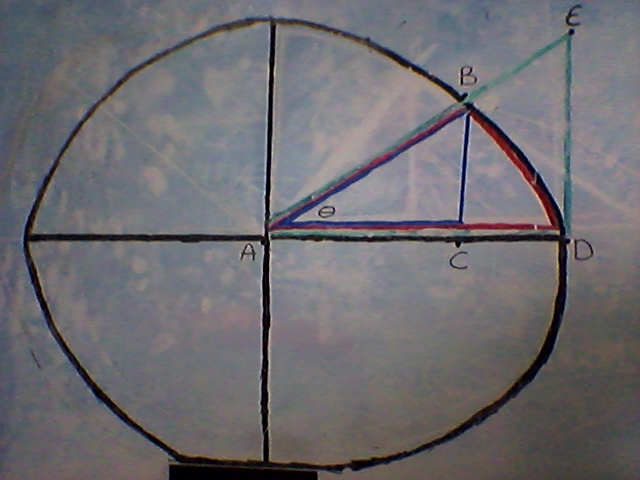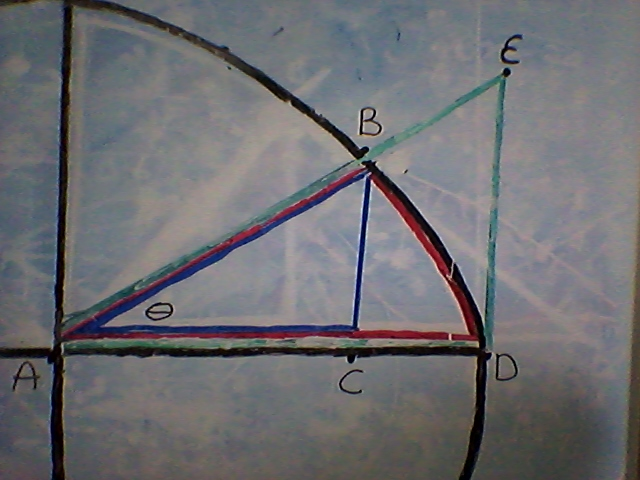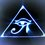# $\displaystyle \lim_{\theta\to 0}\frac { \theta}{sin\theta} = ?$This note is about how to prove $\lim_{\theta\to 0}\frac { \theta}{sin\theta}$ using squeeze theorem.

I have created a diagram to walk us through this procedure. In the diagram there are three shapes we will be addressing. There is the triangle $ABC$ (blue), the sector $ABD$ (red), and the triangle $AED$ (green) which I have drawn on the unit circle.

If you are unfamiliar with the unit circle the main concept is that the unit circle has a radius of one, which is very useful for proving trigonometric identities nonetheless true in this case.

If you are unfamiliar with squeeze theorem It is the idea that if you have three quantities, quantity one, quantity two, and quantity three, where quantity one is less than or equal to quantity two, and quantity three is greater than or equal to quantity two, then at some point quantity one equals quantity three, and quantity two is also equal.

$If$ quantity 1 $\leq$ quantity 2 $\leq$ quantity 3

$and$ quantity 1 $=$ quantity 3

$then$ quantity 1 $=$ quantity 2 $=$ quantity 3

In our case we will be squeezing the area of the three shapes.

area of triangle $ABC$ $\leq$ area of sector $ABD$ $\leq$ area of triangle $AED$

Before we get into the trigonometric definition though I would like to start by pointing out that the angle $\theta$ is unknown. It could be anything. If theta equals zero we have a straight line. If it is 360 degrees we have a circle.Triangle $ABC$

Triangle $ABC$ is a right angled triangle where side length $AB$ is the hypoteneuse which is equal to the radius of the unit circle which is equal to one.

$AB=1$

Side$BC$ is the oppostie side of angle $\theta$.

Side $AC$ is the adjacent side to angle $\theta$

Given that:

$sin\theta = \frac {opposite}{hypoteneuse}$

$sin\theta = \frac {BC}{1}$

$sin\theta = BC$

And given that:

$cos\theta = \frac {adjacent}{hypoteneuse}$

$cos\theta = \frac {AC}{1}$

$cos\theta = AC$

Then the area of the triangle is as follows:

$Area= \frac{1}{2} \times base \times height$

$Area= \frac{1}{2} \times AC \times BC$

$Area= \frac{1}{2} cos\theta sin\theta$

Triangle $AED$

Triangle $AED$ is another right angled triangle where side $AD$ has a length that is equal to the radius of the unit circle which is equal to one.

$AD=1$

Side $AD$ is the adjacent side to angle $\theta$

Side $ED$ is the opposite side to angle $\theta$

Given that:

$tan\theta= \frac{opposite}{adjacent}$

$tan\theta= \frac{ED}{1}$

$tan\theta= ED$

And the area of triangle $AED$ is as follows:

$Area= \frac {1}{2} \times base \times height$

$Area= \frac {1}{2} \times 1 \times ED$

$Area= \frac {1}{2}tan\theta$

$Area= \frac {sin\theta}{2cos\theta}$

($tan\theta= \frac{sin\theta}{cos\theta}$ via the quotient identity.

Sector $ABD$

Sector $ABD$ is a fraction of the unit circle. So we still have our radius $AD$ which is equal to one.

$AD=1$

The angle that makes a circle in radians is $2\pi$ so the fraction of the circle is $\frac{\theta}{2\pi}$.

Then the area of the sector is as follows:

$Area= \frac{\theta}{2\pi} \times \pi \times r^{2}$

$Area= \frac{\theta}{2\pi} \times \pi \times (1)^{2}$

$Area= \frac{\theta}{2\pi} \times \pi \times 1$

$Area= \frac{\theta\times\pi}{2\times\pi}$

$Area= \frac{\theta}{2}$

Now we can substitute these formulas into our inequality and we will have to rearrange a few thing to find the limit we are looking for.

area of triangle $ABC$ $\leq$ area of sector $ABD$ $\leq$ area of triangle $AED$

$\frac{1}{2} cos\theta sin\theta \leq \frac{\theta}{2} \leq \frac {sin\theta}{2cos\theta}$

mulitply everything by $2$

$cos\theta sin\theta \leq \theta\leq \frac {sin\theta}{cos\theta}$

divide everything by $sin \theta$

$cos\theta \leq \frac{\theta}{sin\theta} \leq \frac {1}{cos\theta}$

find the limit of $cos\theta$

$\lim_{\theta\to o} cos\theta \leq \frac{\theta}{sin\theta} \leq \frac {1}{cos\theta}$

$= 1 \leq \frac{\theta}{sin\theta} \leq \frac {1}{1}$

Therefore

$\lim_{\theta\to 0}\frac { \theta}{sin\theta} =1$Note by Brody Acquilano
6 years, 2 months ago

This discussion board is a place to discuss our Daily Challenges and the math and science related to those challenges. Explanations are more than just a solution — they should explain the steps and thinking strategies that you used to obtain the solution. Comments should further the discussion of math and science.

When posting on Brilliant:

• Use the emojis to react to an explanation, whether you're congratulating a job well done , or just really confused .
• Ask specific questions about the challenge or the steps in somebody's explanation. Well-posed questions can add a lot to the discussion, but posting "I don't understand!" doesn't help anyone.
• Try to contribute something new to the discussion, whether it is an extension, generalization or other idea related to the challenge.

MarkdownAppears as
*italics* or _italics_ italics
**bold** or __bold__ bold
- bulleted- list
• bulleted
• list
1. numbered2. list
1. numbered
2. list
Note: you must add a full line of space before and after lists for them to show up correctly
paragraph 1paragraph 2

paragraph 1

paragraph 2

[example link](https://brilliant.org)example link
> This is a quote
This is a quote
    # I indented these lines
# 4 spaces, and now they show
# up as a code block.

print "hello world"
# I indented these lines
# 4 spaces, and now they show
# up as a code block.

print "hello world"
MathAppears as
Remember to wrap math in $$ ... $$ or $ ... $ to ensure proper formatting.
2 \times 3 $2 \times 3$
2^{34} $2^{34}$
a_{i-1} $a_{i-1}$
\frac{2}{3} $\frac{2}{3}$
\sqrt{2} $\sqrt{2}$
\sum_{i=1}^3 $\sum_{i=1}^3$
\sin \theta $\sin \theta$
\boxed{123} $\boxed{123}$

Sort by:

It's an interesting approach, going through proving that using geometry!

I was thinking of a calculus-based proof:

If we prove, using basic calculus ( Variation -derivatives ...) , that:

$\displaystyle \sin x < x < \tan x ; \forall x>0$

$\displaystyle \tan x < x < \sin x ; \forall x<0$,

we could also conclude the value of the limit to be 1, after calculating both handed limits.

But proving that using geometry was exciting! Cheers!!

- 6 years, 2 months ago

Cheers.

- 6 years, 2 months ago

It seems to me that you have merely shown that

$\sin \theta \leq \theta \leq \tan \theta.$

How does that allow us to conclude what $\lim \frac{ \sin \theta } { \theta}$ is?

Staff - 6 years, 2 months ago

Sorry it's finished now. Hope I did alright.

- 6 years, 2 months ago

Right, that's the final step that's needed to draw the conclusion.

To get such "complicated" expressions to display nicely while inline, you will need to use \displaystyle, which forces the superscript / subscript, which increases out the line spacing (and hence making it uglier).

IE \displaystyle \lim_{x \rightarrow 0 } gives us $\displaystyle \lim_{x \rightarrow 0 }$

Staff - 6 years, 2 months ago

Thanks I will have to try that!

- 6 years, 2 months ago

I couldn't get the $\to 0$ to go under the $\lim$ that was one thing I have to fix probably.

- 6 years, 2 months ago

By observing the given problem limit extends to 0 means the answer might be infinite(or)zero

- 6 years, 2 months ago